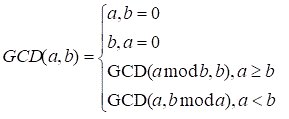bolt
Try our new interface for solving problems
Problems

# GCD of two numbers

Time limit 1 second
Memory limit 128 MiB

Find the GCD (greatest common divisor) of two nonnegative integers.## Input data

Two integers a and b\:(a, b \le 2 \cdot 10^9).

## Output data

Print the GCD of a and b.

## Examples

Input example #1
42 24

Output example #1
6